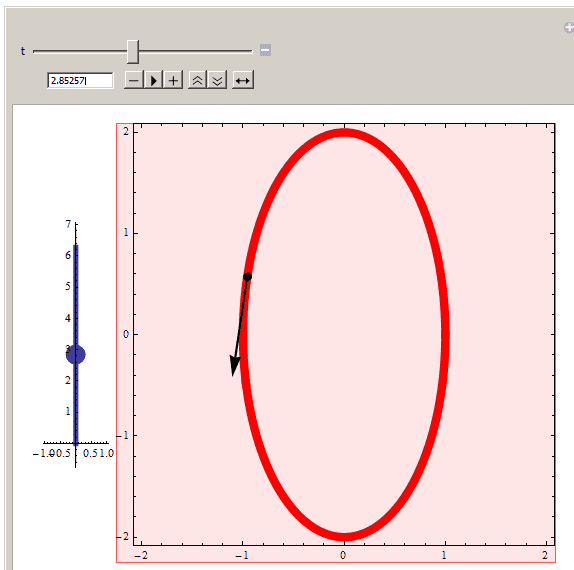# Truly Bizarre - The unit tangent and unit normal vectors aren't orthogonal!

jdinatale
OK, this looks like a differential geometry problem, which it is, but at the end of the day I am trying to figure out why the unit normal and unit tangent vectors to a curve aren't orthogonal, so even if you don't know about DG, please respond.Obviously the two choices for E_1 and E_2 are the unit normal and unit tangent vectors to the curve.

Using Mathematica...

alpha[t_] := {Cos[t], 2 Sin[t]};

alphaprime[t_] := {-Sin[t], 2 Cos[t]};

alphaprimeprime[t_] := {-Cos[t], -2 Sin[t]};

unittangentvector[t_] := alphaprime[t] / Norm[alphaprime[t]];

unitnormalvector[t_] := alphaprimeprime[t] / Norm[alphaprimeprime[t]];

Or by hand...

$\alpha(t) = {Cos(t), 2Sin(t)}$
$\alpha'(t) = {-Sin(t), 2Cos(t)}$
$\alpha''(t) = {-Cos(t), -2Sin(t)}$

However, graphically, the unit tangent and unit normal vectors are far from perpendicular on this curve!Here is my mathematica code

VFieldOnCurve2D[dominterval_, CurveEq_, FrameField_, CodomainBox_,
Size_] :=
Module[{a2, b2, Content, IS, DomainPieces, DomainPiece1,
DomainPiece2, CodomainCenter, CodomainWidth, len, EE1, EE2,
ImagePieces, ImagePiece0, ImagePiece1, ImagePiece2},
{a2, b2} = dominterval;
IS = 300;
Content = Mapping12Content[dominterval, CurveEq];
DomainPiece1 = Content[];
DomainPiece2[t_] := Points2D[{{0, t}}, .3];
DomainPieces[t_] :=
Show[DomainPiece1, DomainPiece2[t], ImageSize -> IS/4];

{CodomainCenter, CodomainWidth} = CodomainBox;
len = Length[FrameField];
If[len == 2, EE1 = FrameField[];
EE2 = FrameField[], {EE1} = FrameField];

ImagePiece0 = EmptySpace2DXCenter[CodomainCenter, CodomainWidth];
ImagePiece1 = Content[];
ImagePiece2[t_] :=
If[len == 2, {Vec[CurveEq[t], EE1[t]], Vec[CurveEq[t], EE2[t]]},
Vec[CurveEq[t], EE1[t]] ];
ImagePieces[t_] :=
Show[ImagePiece0, ImagePiece1, ImagePiece2[t], ImageSize -> Size];

t0 = (a2 + b2)/2;
Manipulate[
Row[{DomainPieces[t], ImagePieces[t]}], {{t, t0, "t"}, a2, b2},
SaveDefinitions -> True]

]

alpha[t_] := {Cos[t], 2 Sin[t]};

alphaprime[t_] := {-Sin[t], 2 Cos[t]};

alphaprimeprime[t_] := {-Cos[t], -2 Sin[t]};

unittangentvector[t_] := alphaprime[t] / Norm[alphaprime[t]];

unitnormalvector[t_] := alphaprimeprime[t] / Norm[alphaprimeprime[t]];

E1[t_] = unitnormalvector[t];
E2[t_] = unittangentvector[t];

DomainInterval = {0, 2 \[Pi]};
initvalue = 0;
CodomainBox = {Origin2D, 2};
Size = 400;
VFieldOnCurve2D[DomainInterval, alpha, {E1, E2}, CodomainBox, Size]

## Answers and Replies

Homework Helper
The unit normal isn't alphaprimeprime[t] / Norm[alphaprimeprime[t]]. It's the derivative of the UNIT tangent vector divided by the norm of the derivative of the UNIT tangent vector. alphaprime isn't UNIT.

Last edited:
jdinatale
The unit normal isn't alphaprimeprime[t] / Norm[alphaprimeprime[t]]. It's the derivative of the UNIT tangent vector divided by the norm of the derivative of the UNIT tangent vector. alphaprime isn't UNIT.

Thanks, and that would seem to do the trick, but mathematica is hating me right now. Any ideas what's going on? Here is the newly defined unit normal vector

alpha[t_] := {Cos[t], 2 Sin[t]};

alphaprime[t_] := {-Sin[t], 2 Cos[t]};

alphaprimeprime[t_] := {-Cos[t], -2 Sin[t]};

unittangentvector[t_] := alphaprime[t] / Norm[alphaprime[t]];

unitnormalvector[t_] :=
unittangentvector'[t] / Norm[unittangentvector'[t] ];

E1[t_] = unitnormalvector[t];
E2[t_] = unittangentvector[t];Implementation

VFieldOnCurve2D[dominterval_, CurveEq_, FrameField_, CodomainBox_,
Size_] :=
Module[{a2, b2, Content, IS, DomainPieces, DomainPiece1,
DomainPiece2, CodomainCenter, CodomainWidth, len, EE1, EE2,
ImagePieces, ImagePiece0, ImagePiece1, ImagePiece2},
{a2, b2} = dominterval;
IS = 300;
Content = Mapping12Content[dominterval, CurveEq];
DomainPiece1 = Content[];
DomainPiece2[t_] := Points2D[{{0, t}}, .3];
DomainPieces[t_] :=
Show[DomainPiece1, DomainPiece2[t], ImageSize -> IS/4];

{CodomainCenter, CodomainWidth} = CodomainBox;
len = Length[FrameField];
If[len == 2, EE1 = FrameField[];
EE2 = FrameField[], {EE1} = FrameField];

ImagePiece0 = EmptySpace2DXCenter[CodomainCenter, CodomainWidth];
ImagePiece1 = Content[];
ImagePiece2[t_] :=
If[len == 2, {Vec[CurveEq[t], EE1[t]], Vec[CurveEq[t], EE2[t]]},
Vec[CurveEq[t], EE1[t]] ];
ImagePieces[t_] :=
Show[ImagePiece0, ImagePiece1, ImagePiece2[t], ImageSize -> Size];

t0 = (a2 + b2)/2;
Manipulate[
Row[{DomainPieces[t], ImagePieces[t]}], {{t, t0, "t"}, a2, b2},
SaveDefinitions -> True]

]

alpha[t_] := {Cos[t], 2 Sin[t]};

alphaprime[t_] := {-Sin[t], 2 Cos[t]};

alphaprimeprime[t_] := {-Cos[t], -2 Sin[t]};

unittangentvector[t_] := alphaprime[t] / Norm[alphaprime[t]];

unitnormalvector[t_] :=
unittangentvector'[t] / Norm[unittangentvector'[t] ];

E1[t_] = unitnormalvector[t];
E2[t_] = unittangentvector[t];

DomainInterval = {0, 2 \[Pi]};
initvalue = 0;
CodomainBox = {Origin2D, 2};
Size = 400;
VFieldOnCurve2D[DomainInterval, alpha, {E1, E2}, CodomainBox, Size]

Homework Helper
Not really, sorry. It looks right. But I haven't done Mathematica since other people stopped paying for it for me. It's really expensive and unfree. So I can't test that in any detail.

jdinatale
Not really, sorry. It looks right. But I haven't done Mathematica since other people stopped paying for it for me. It's really expensive and unfree. So I can't test that in any detail.

Well, at least theoretically, would you agree that my choice of E1 and E2 provide a frame field on the curve?